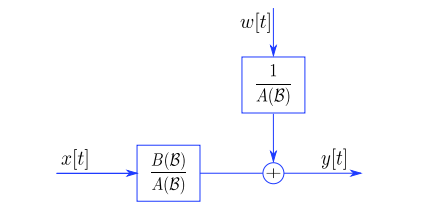# General Techniques for Battery Parameter Identification

There are a number of ways to describe battery physics like Physical model, Equivalent circuit model, Fractional order model, Blackbox model, etc. But to choose which model will best fit your battery accumulator is quite critical.

The simplest model to adapt for battery physics is the Equivalent circuit model because its numerical simplicity and understanding are quite simple as compared to other existing models. There are different ways to configure the ECM model using different RC pair configurations. Here we discuss the Thevenin model to describe battery physics which is quite a decent model to study. We can use more RC pairs in the Thevenin model depending on the requirement but to make this article simple we are using the 1RC model here.

Thevenin Model (1-RC Model)

Open circuit model (OCV) of the cell is described by the voltage source component in the above circuit and the battery’s internal resistance and diffusion are described by R_int and Rp & Cp component respectively.

Dynamic voltage can be expressed as: -

where OCV = Open Circuit Voltage

Ut = Terminal voltage

R0= Internal resistance

R1= Diffusion resistance

I= Applied current

For Battery state estimation, it is very critical to find the parameters which described good battery physics. The good point of this model is that we don’t need to dissect the cell to find the parameters, it's a non-destructive method which makes this model universally acceptable. There are different numbers if ways to find these RC parameters like OKID-ERA, State-Space, Subspace, transfer function, etc. but here we are going to discuss how we can estimate those parameters using the transfer function system ID

The transfer function model can be described in many ways depending on the noise and disturbance. For measurement noise in the system we use Output Error model and for process disturbance in the system we use ARMAX model and for both noises, we use Box-Jenkins models, and for the less complex system, we as a Grinntech Motors sometimes use ARX model for real-time parameter estimation which is very much related to output error model to tackle the voltage sensor noise and here we are going to discuss the ARX model which is less numerically complex and quite simple to implement.

ARX model is described asPicture Credit: - Paper Title, Statistical Time Series Methods for Vibration-Based Structural Health Monitoring.

where w is measurement noise, x is applied current and y is your terminal voltage.

ARX Model

ARX Model equation is expressed as: -

y(k) = G(q)*u(k n) + w(k),

G(q) = B(q)/ A(q)

A(q) = 1 + a1q−1 + a2q−1 + ··· + akq−n

B(q) = b + b1q−1 + b2q−1 + ··· + bkq−n

The battery equation can be expressed like an ARX model equation to find the regressor vector(G) and output vector(Y) which can be expressed as

Y=G*X

We do the slow rate charge-discharge and dynamic testing like the UDDS cycle, HPPC cycle, at Grinntech Motors facilities to acquire enough data to understand the relationship between SOC-OCV and dynamic behavior of the cell concerning dynamic load current as expressed in the dynamic voltage equation(Eqn-1).

Once you choose the best fit model for your system just like we did here use the ARX model for your system and do the bilinear transformation to find the relationship between the regressor vector, output vector, and parameter vector.

The below equation is expressed for 1RC Transfer function model for your reference

Once we have enough test data we can form a regressor and output matrix similar to the matrix mentioned in the above equation, the above equation varies based on the number of RC pair you want to identify. Once you form the regressor matrix and output matrix we can easily identify the parameters(X) using the least square method. The least-square method which is based on linear algebra projection is one of the most common methods to identify the unknown parameter.